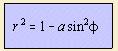# hippopede

## quartic

last updated: 2005-04-23Hippopede means literally 'foot of a horse'; the curve is also known as the horse fetter.
Because of the work done by J. Booth (1810-1878), the curve is also named the curve of Booth.

The curve has three forms:

For a < 1, the curve is the oval of Booth.
For a > 1, the curve is the lemniscate of Booth.
When the parameter a has the value 2, the curve is the lemniscate of Bernoulli.

Proclus
(75 BC) and Eudoxus were the first to investigate the curve.
After Proclus the curve has been named hippopede of Proclus.

In Cartesian coordinates the curve has the form: (x2 + y2)2 = b x2 + y2 with b = 1 - a.
And we see that the curve is a bicircular (rational) quartic.

The hippopede can be seen as:

• the cissoid of two circles of equal size
• a Watt's curve for which the length of the rod and the distance between the circles are equal
• a special case of the spiric section for which the plane is tangent to the interior of the torus